In this paper the tsfknn package for time series forecasting using KNN regression is described. The package allows, with only one function, to specify the KNN model and to generate the forecasts. The user can choose among different multi-step ahead strategies and among different functions to aggregate the targets of the nearest neighbors. It is also possible to consult the model used in the prediction and to obtain a graph including the forecast and the nearest neighbors used by KNN.

# 1 Introduction

Time series forecasting has been performed traditionally using statistical methods such as ARIMA models or exponential smoothing. However, the last decades have witnessed the use of computational intelligence techniques to forecast time series. Although artificial neural networks is the most prominent machine learning technique used in time series forecasting, other approaches, such as Gaussian Process or KNN, have also been applied. Compared with classical statistical models, computational intelligence methods exhibit interesting features, such as their nonlinearity or the lack of an underlying model, that is, they are non-parametric.

Statistical methodologies for time series forecasting are present in CRAN as excellent packages. For example, the forecast package includes implementations of ARIMA, exponential smoothing, the theta method or basic techniques, such as the naive approach, that can be used as benchmark methods. On the other hand, although a great variety of computational intelligence approaches for regression are available in R (see, for example, the caret package), these approaches cannot be directly applied to time series forecasting. Fortunately, some new packages are filling this gap. For example, the nnfor package or the nnetar function from the forecast package allow to predict time series using artificial neural networks.

KNN is a very popular algorithm used in classification and regression. This algorithm simply stores a collection of examples. Each example consists of a vector of features (describing the example) and its associated class (for classification) or numeric value (for prediction). Given a new example, KNN finds its k most similar examples (called nearest neighbors), according to a distance metric (such as the Euclidean distance), and predicts its class as the majority class of its nearest neighbors or, in the case of regression, as an aggregation of the target values associated with its nearest neighbors. In this paper we describe the tsfknn R package for univariate time series forecasting using KNN regression.

The rest of the paper is organized as follows. Section 2 explains how KNN regression can be applied in a time series forecasting context using the tsfknn package. In Section 3 the different multi-step ahead strategies implemented in our package are explained. Section 4 discusses some additional feature of our package. In Section 5 describes how the forecast accuracy of a KNN model can be assessed using a rolling origin evaluation. Finally, Section 6 draws some conclusions.

# 2 Time series forecasting with KNN regression

In this section we explain how KNN regression can be applied to forecast time series. To this end, we will use some functionality of the package tsfknn. Let us start with a simple time series: $$t = \{ 1, 2, 3, 4, 5, 6, 7, 8 \}$$ and suppose that we want to predict its next future value. First, we have to determine how the KNN examples are built, that is, we have to decide what are the features and the targets associated with an example. The target of an example is a value of the time series and its features are lagged values of the target. For example, if we use lags 1-2 as features, the examples associated with the time series $$t$$ are:

Features Target
1, 2 3
2, 3 4
3, 4 5
5, 6 7
6, 7 8

In our package, you can consult the examples associated with a KNN model used for time series forecasting with the knn_examples function:

library(tsfknn)
pred <- knn_forecasting(ts(1:8), h = 1, lags = 1:2, k = 2, transform = "none")
knn_examples(pred)
##      Lag2 Lag1 H1
## [1,]    1    2  3
## [2,]    2    3  4
## [3,]    3    4  5
## [4,]    4    5  6
## [5,]    5    6  7
## [6,]    6    7  8

Before consulting the examples, you have to build the model. This is done with the function knn_forecasting that builds a model associated with a time series and uses the model to predict the future values of the time series. Let us see the main arguments of this function:

timeS : the time series to be forecast.

h : the forecast horizon, that is, the number of future values to be predicted.

lags : an integer vector indicating the lagged values of the target used as features in the examples (for instance, 1:2 means that lagged values 1 and 2 should be used).

k : the number of nearest neighbors used by the KNN model.

knn_forecasting is very handy because, as commented above, it builds the KNN model and then uses the model to predict the time series. This function returns a knnForecast object with information of the model and its prediction. As we have seen above, you can use the function knn_examples to see the examples associated with the model. You can also consult the prediction or get a plot through the knnForecast object:

pred$prediction ## Time Series: ## Start = 9 ## End = 9 ## Frequency = 1 ##  7.5 plot(pred)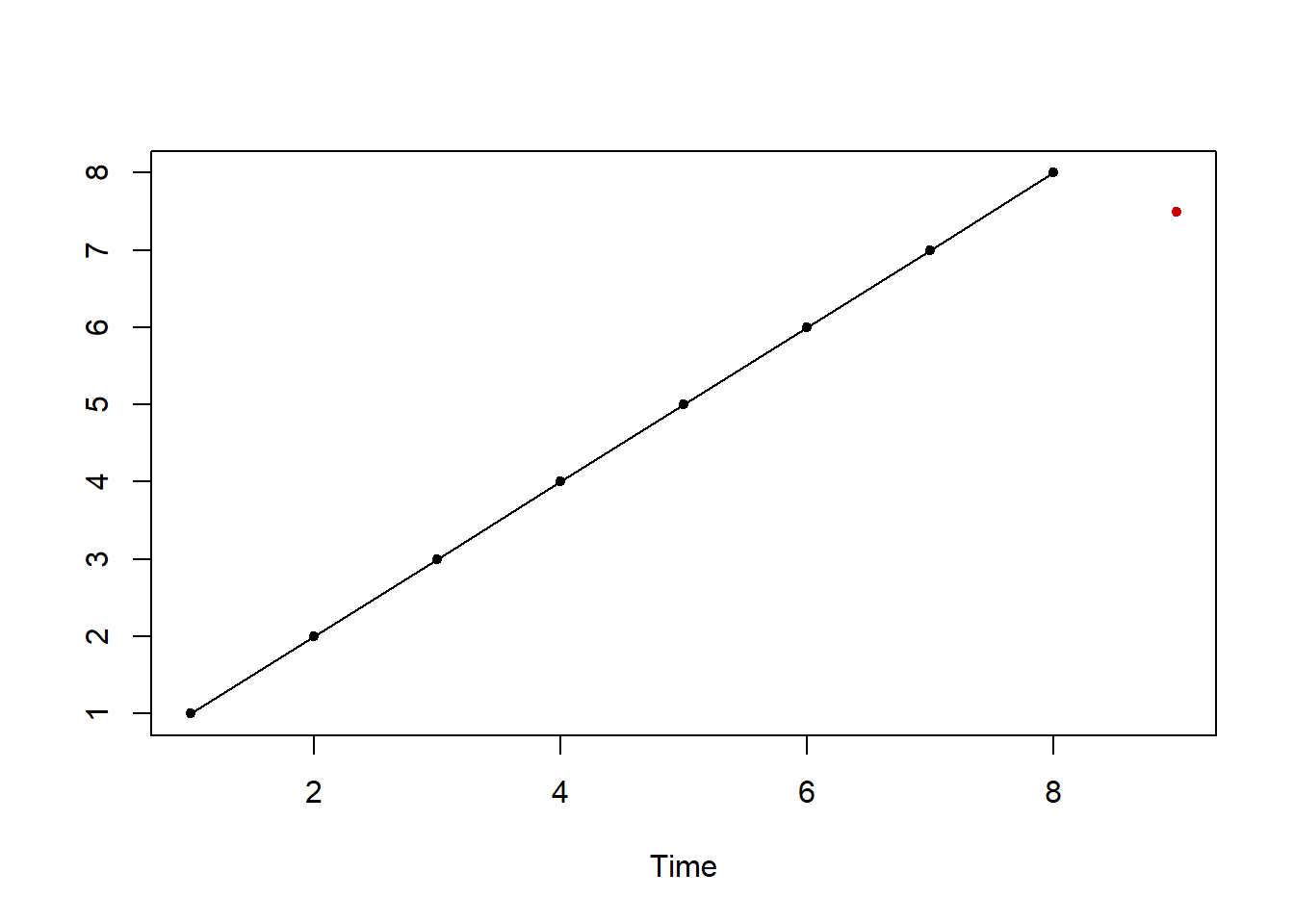You can also consult how the prediction was made. That is, you can consult the instance whose target was predicted and its nearest neighbors. This information is obtained with the nearest_neighbors function applied to a knnForecast object: nearest_neighbors(pred) ## [] ## []$instance
## Lag 2 Lag 1
##     7     8
##
## []$nneighbors ## Lag 2 Lag 1 H1 ## 1 6 7 8 ## 2 5 6 7 Because we have used lags 1-2 as features, the features associated with the next future value of the time series are the last two values of the time series (vector $$[7, 8]$$). The two most similar examples (nearest neighbors) of this instance are vectors $$[6, 7]$$ and $$[5, 6]$$, whose targets (8 and 7) are averaged to produce the prediction 7.5. You can obtain a nice graph including the instance, its nearest neighbors and the prediction: library(ggplot2) autoplot(pred, highlight = "neighbors")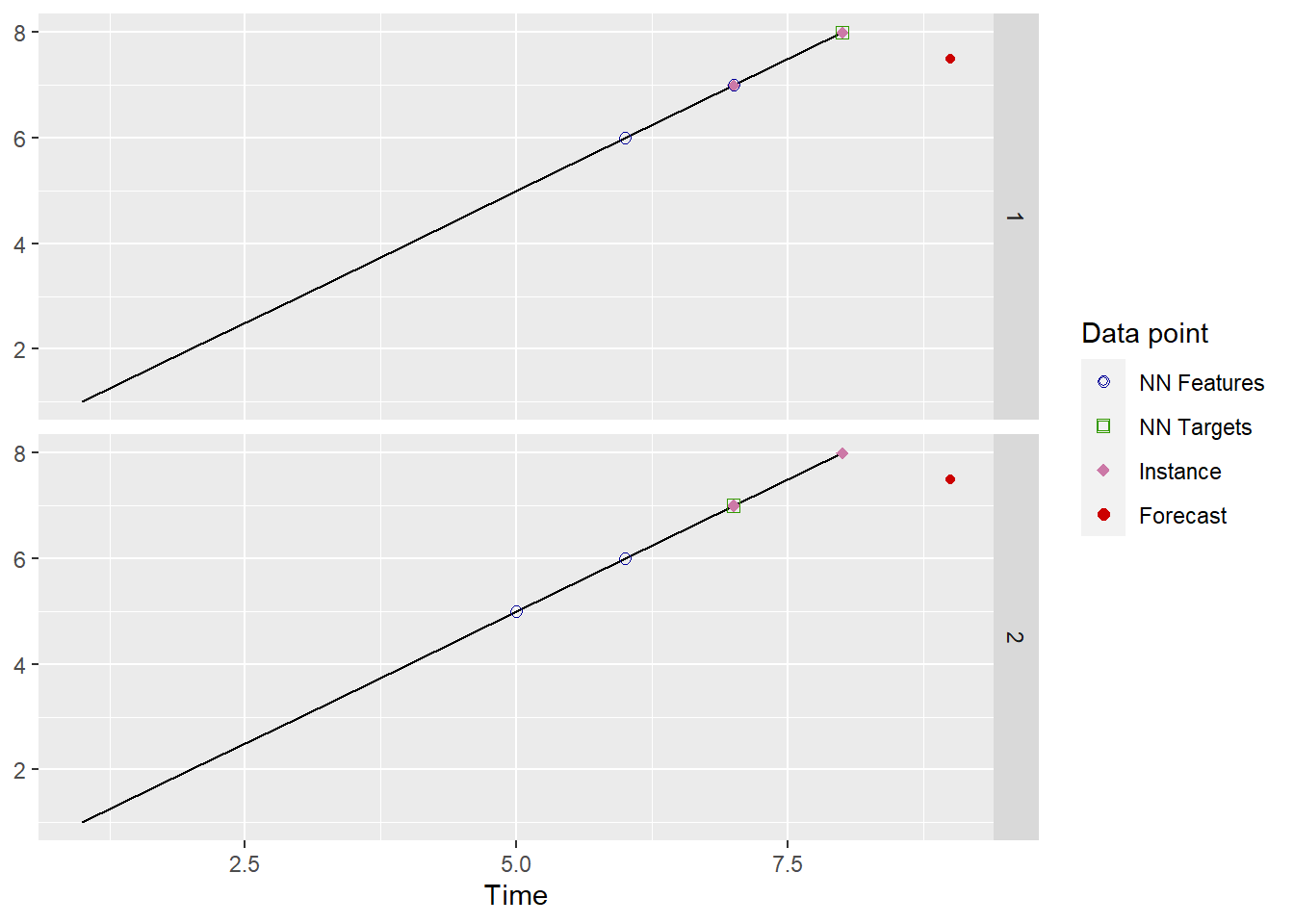As can be observed, each nearest neighbor has been plotted in a different plot (you can also select to get all the nearest neighbors in the same plot). The neighbors in the plots are sorted according to their distance to the instance, being the neighbor in the top plot the nearest neighbor. By the way, this artificial example of a time series with a constant linear trend illustrates the fact that KNN is not suitable for predicting time series with a global trend. This is because KNN predicts an aggregation of historical values of the time series. Therefore, in order to predict a time series with global trend some detrending scheme should be used. To recapitulate, because we use univariate time series, to specify a KNN model in our package you have to set: • the lags used to build the KNN examples. They determine the lagged values used as features or autoregressive explanatory variables. • k: the number of nearest neighbors used in the prediction. # 3 Multi-step ahead strategies In the previous section we have seen an example of one-step ahead prediction with KNN. Nonetheless, it is very common to forecast more than one value into the future. To this end, a multi-step ahead strategy has to be chosen. Our package implements two common strategies: the MIMO approach and the recursive or iterative approach (when only one future value is predicted both strategies are equivalent). Let us see how they work. ## 3.1 The Multiple Input Multiple Output strategy This strategy is commonly applied with KNN and it is characterized by the use of a vector of target values. The length of this vector is equal to the number of periods to forecast. For example, let us suppose that we are working with a time series of hourly electricity demand and we want to forecast the demand for the next 24 hours. In this situation, a good choice for the lags would be 1-24, that is, the demand of 24 consecutive hours. If the MIMO strategy is chosen, then an example consists of: • a feature vector with the demand of 24 consecutive hours and • a target vector with the demand in the next 24 consecutive hours (after the 24 hours of the feature vector). The new instance would be the demand in the last 24 hours of the time series. This way, we would look for the demands most similar to the last 24 hours in the time series and we would predict an aggregation of their subsequent 24 hours. In the next example we predict the next 12 months of a monthly time series using the MIMO strategy: pred <- knn_forecasting(USAccDeaths, h = 12, lags = 1:12, k = 2, msas = "MIMO") autoplot(pred, highlight = "neighbors", faceting = FALSE)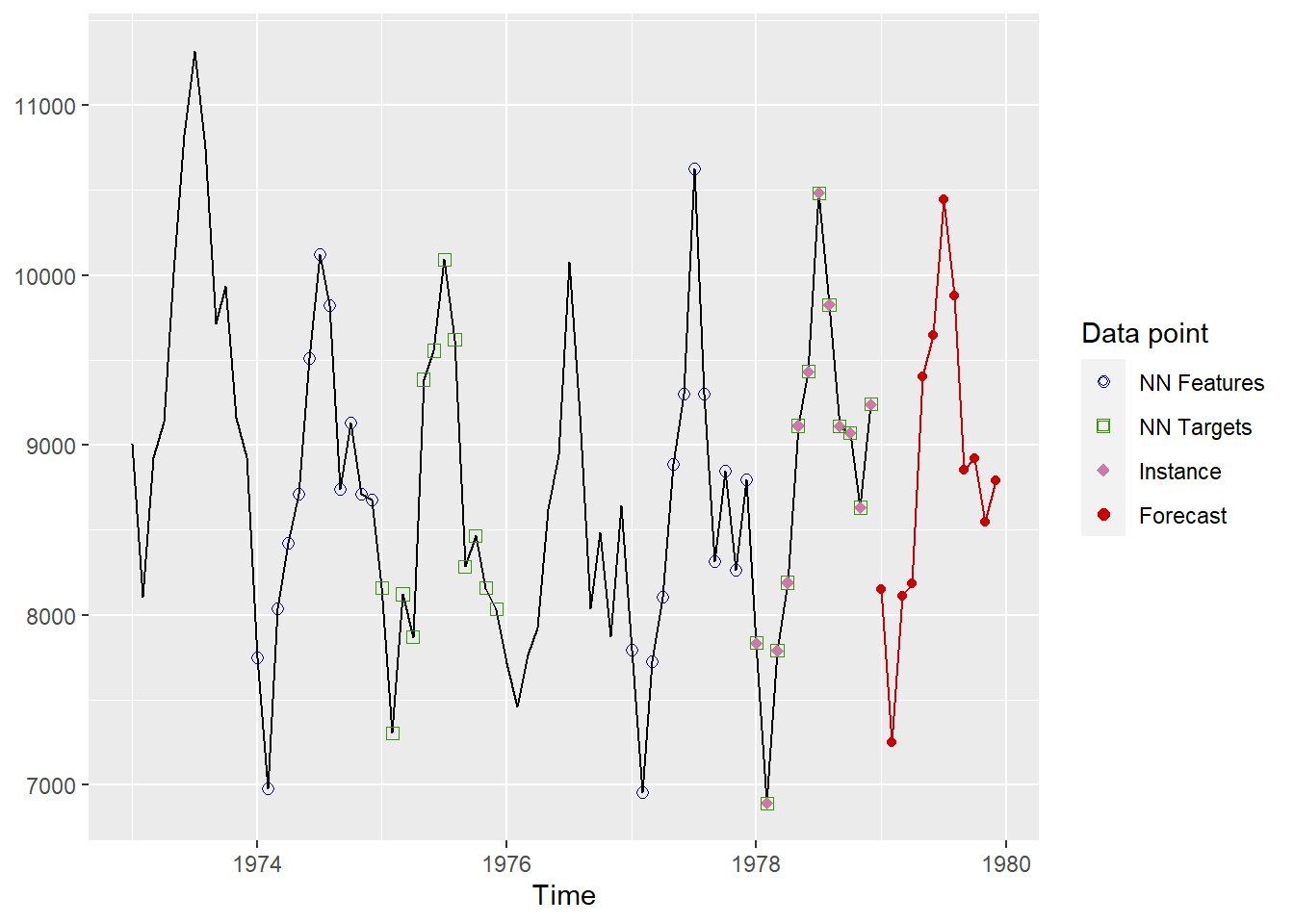The prediction is the average of the target vectors of the two nearest neighbors. As can be observed, we have chosen to see all the nearest neighbors in the same graph. Because we are working with a monthly time series, we have thought that lags 1-12 are a suitable choice for selecting the features of the examples. In this case, the last 12 values of the time series are the new instance whose target has to be predicted. The two sequences of 12 consecutive values most similar to this instance are found (in blue) and their subsequent 12 values (in green) are averaged to obtain the prediction (in red). ## 3.2 The recursive strategy The recursive or iterative strategy is the approach used by ARIMA or exponential smoothing to forecast several periods. Basically, a model that only forecasts one-step ahead is used, so that the model is applied iteratively to forecast all the future periods. When historical observations to be used as features of the new instance are unavailable, previous predictions are used instead. Because the recursive strategy uses a one-step ahead model, this means that, in the case of KNN, the target of an example only contains one value. For instance, let us see how the recursive strategy works with the following example in which the next two future quarters of a quarterly time series are predicted: timeS <- window(UKgas, start = c(1976, 1)) pred <- knn_forecasting(timeS, h = 2, lags = 1:4, k = 2, msas = "recursive") autoplot(pred, highlight = "neighbors")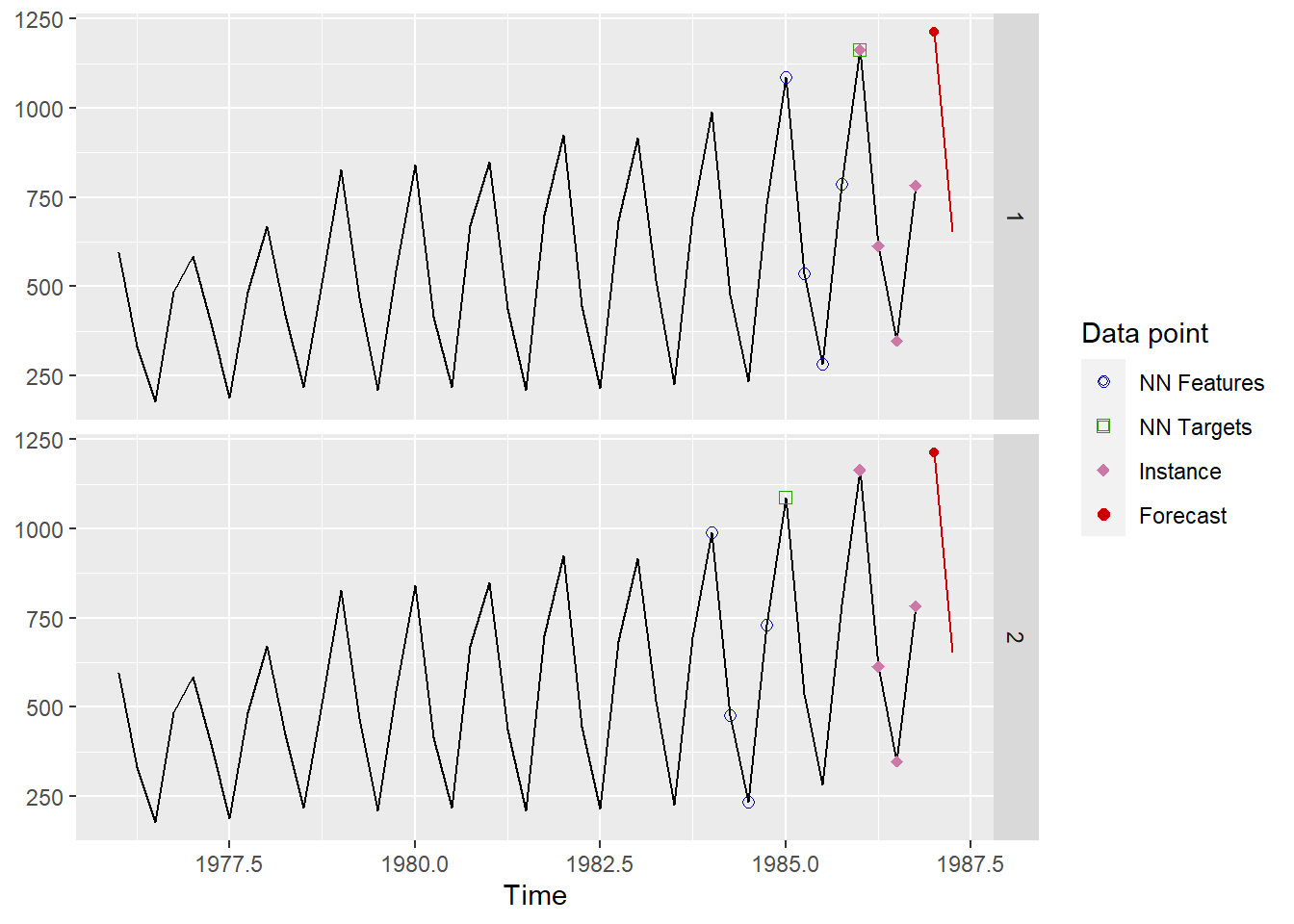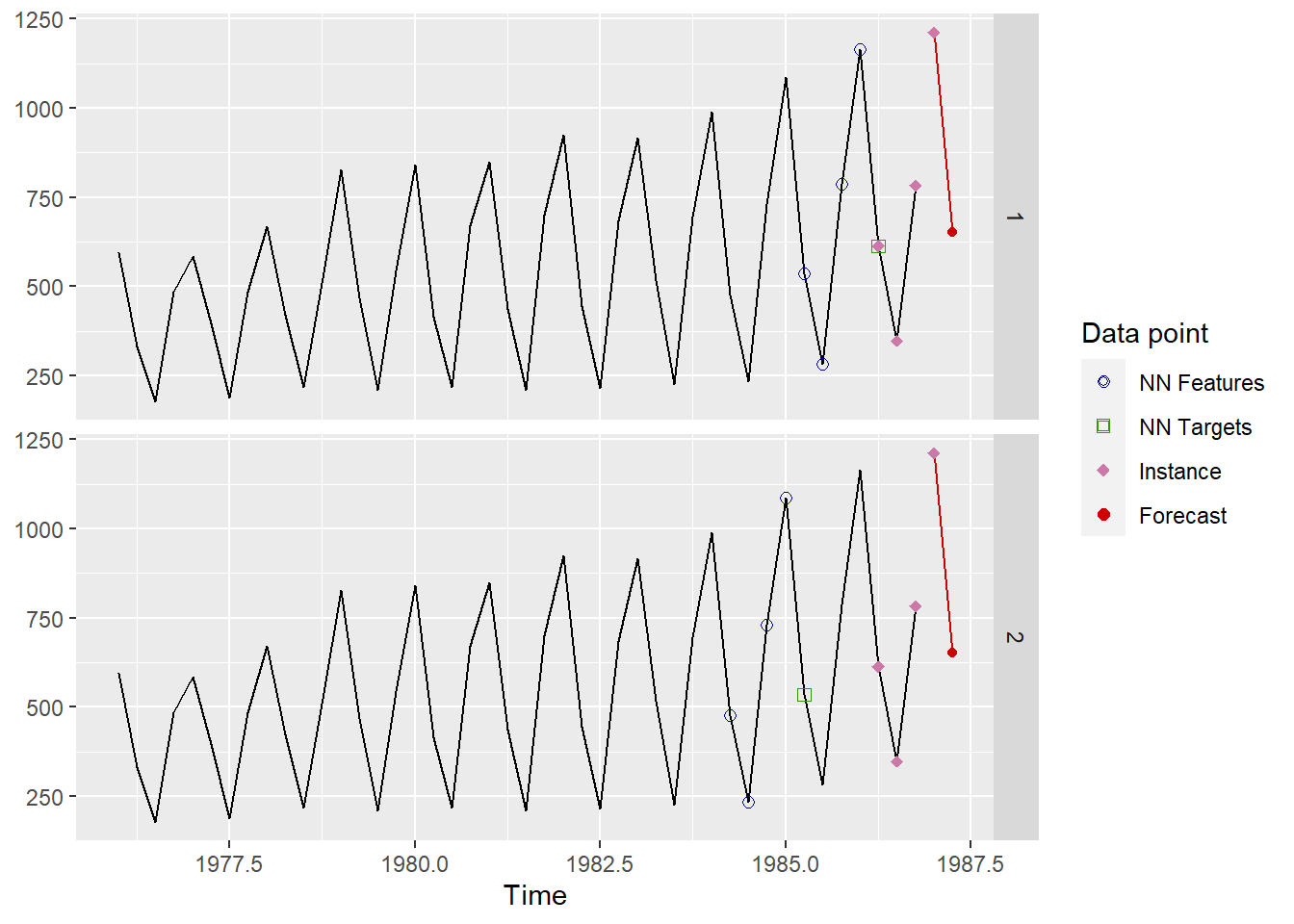In this example we have used lags 1-4 to specify the features of an example. To predict the first future point the last 4 values of the time series are used as “its features”. To predict the second future point “its features” are the last three values of the time series and the prediction for the first future point. In the top graph the prediction for the first future point can be seen and in the bottom graph the prediction for the second point. # 4 Additional features In this section several additional features of our package are described. ## 4.1 Combination and distance function By default, the targets of the different nearest neighbors are averaged. However, it is possible to combine the targets using other aggregation functions. Currently, our package allows to choose among the mean, the median and a weighted mean using the cb parameter of the knn_forecasting function. Regarding the distance function applied to compute the nearest neighbors, our package uses the Euclidean distance, although we can implement other distance metrics in the future. ## 4.2 Combining several models with different k parameters In order to specify a KNN model the user has to select, among other things, the value of the k parameter. Several strategies can be used to choose this value. A first, fast, straightforward solution is to use some heuristic (it is recommended setting k to the square root of the number of training examples). Other approach is to select k using an optimization tool on a validation set. k should minimize a forecast accuracy measure. The optimization strategy is very time consuming. A third strategy is to use several KNN models with different k values. Each KNN model generates its forecasts and the forecasts of the different models are averaged to produce the final forecast. This strategy is based on the success of model combination in time series forecasting. This way, the use of a time consuming optimization tool is avoided and the forecasts are not based on an unique, heuristic k value. In our package you can use of this strategy specifying a vector of k values: pred <- knn_forecasting(ldeaths, h = 12, lags = 1:12, k = c(2, 4)) pred$prediction
##           Jan      Feb      Mar      Apr      May      Jun      Jul      Aug
## 1980 2736.719 2901.029 2610.875 2098.239 1765.176 1515.711 1402.958 1305.580
##           Sep      Oct      Nov      Dec
## 1980 1211.597 1428.876 1575.126 2256.334
plot(pred)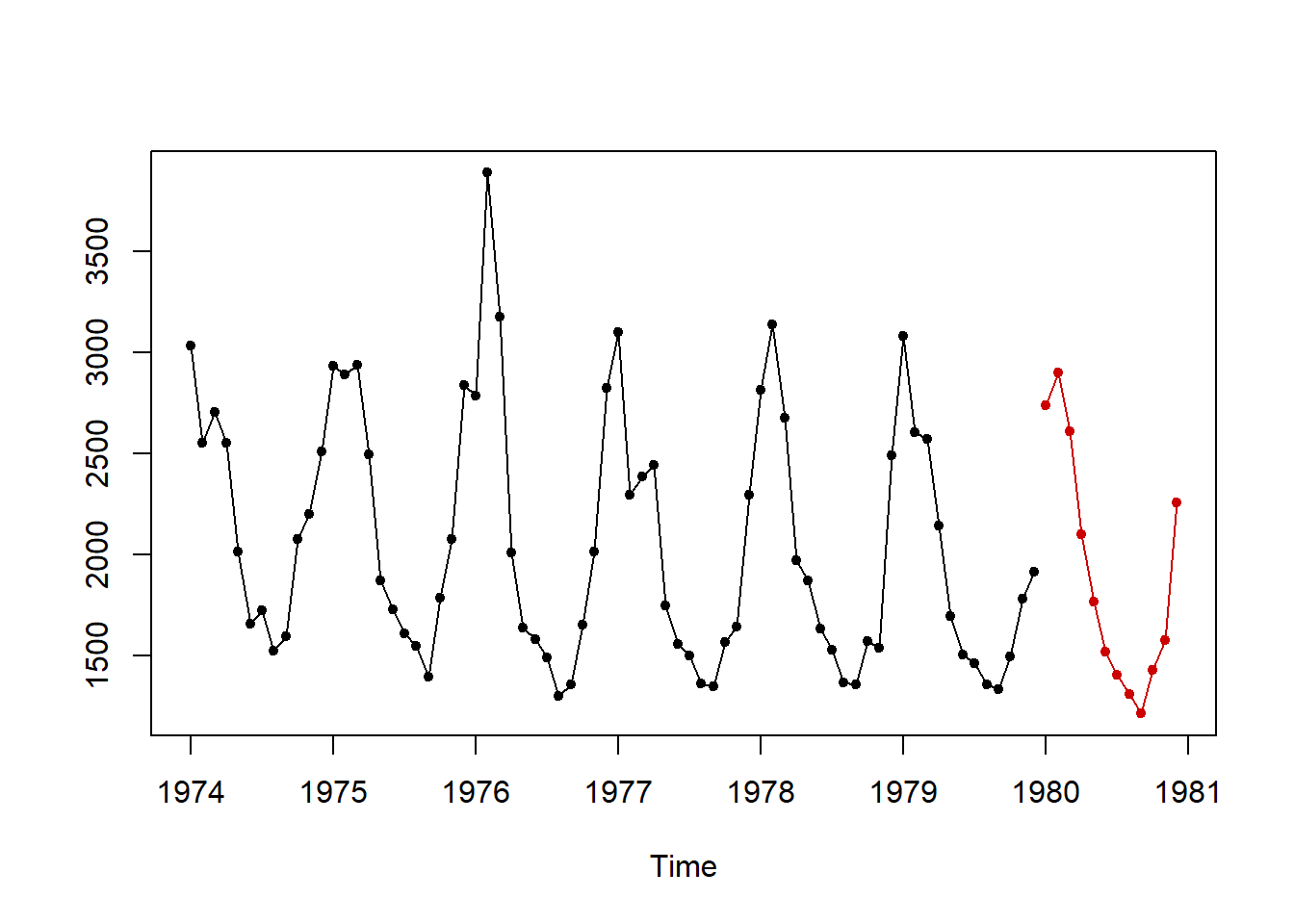## 4.3 Forecasting time series with a trend

KNN is not suitable for forecasting a time series with a trend. The reason is simple, KNN predicts an average of historical values of the time series, so it cannot predict correctly values out of the range of the time series. In your time series has a trend we recommend using the parameter transform to transform the training samples. Use the value "additive"if the trend is additive or "multiplicative" for exponential time series:

set.seed(5)
timeS <- ts(1:10 + rnorm(10, 0, .2))
pred <- knn_forecasting(timeS, h = 3, transform = "none")
plot(pred)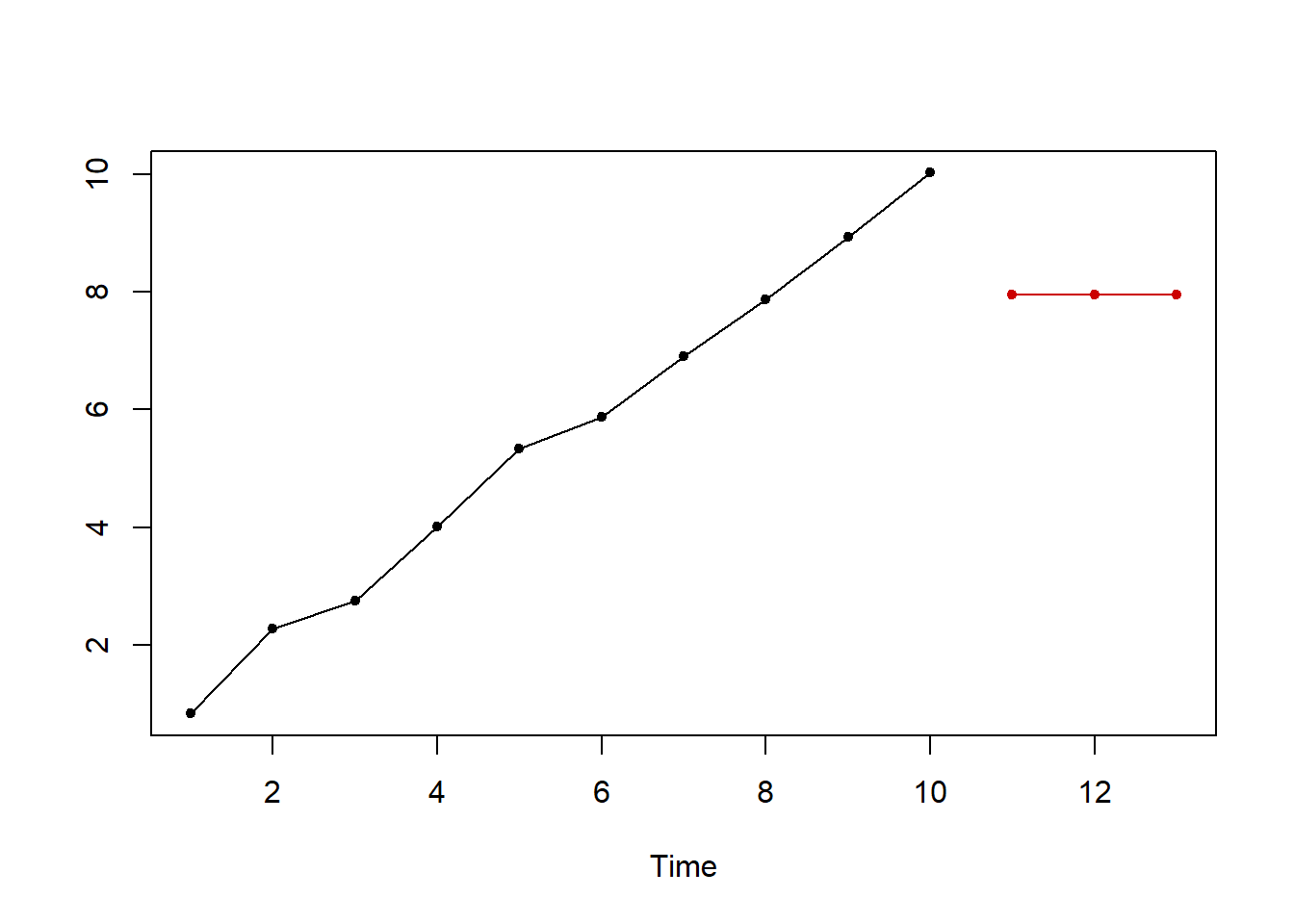pred2 <- knn_forecasting(timeS, h = 3, transform = "additive")
plot(pred2)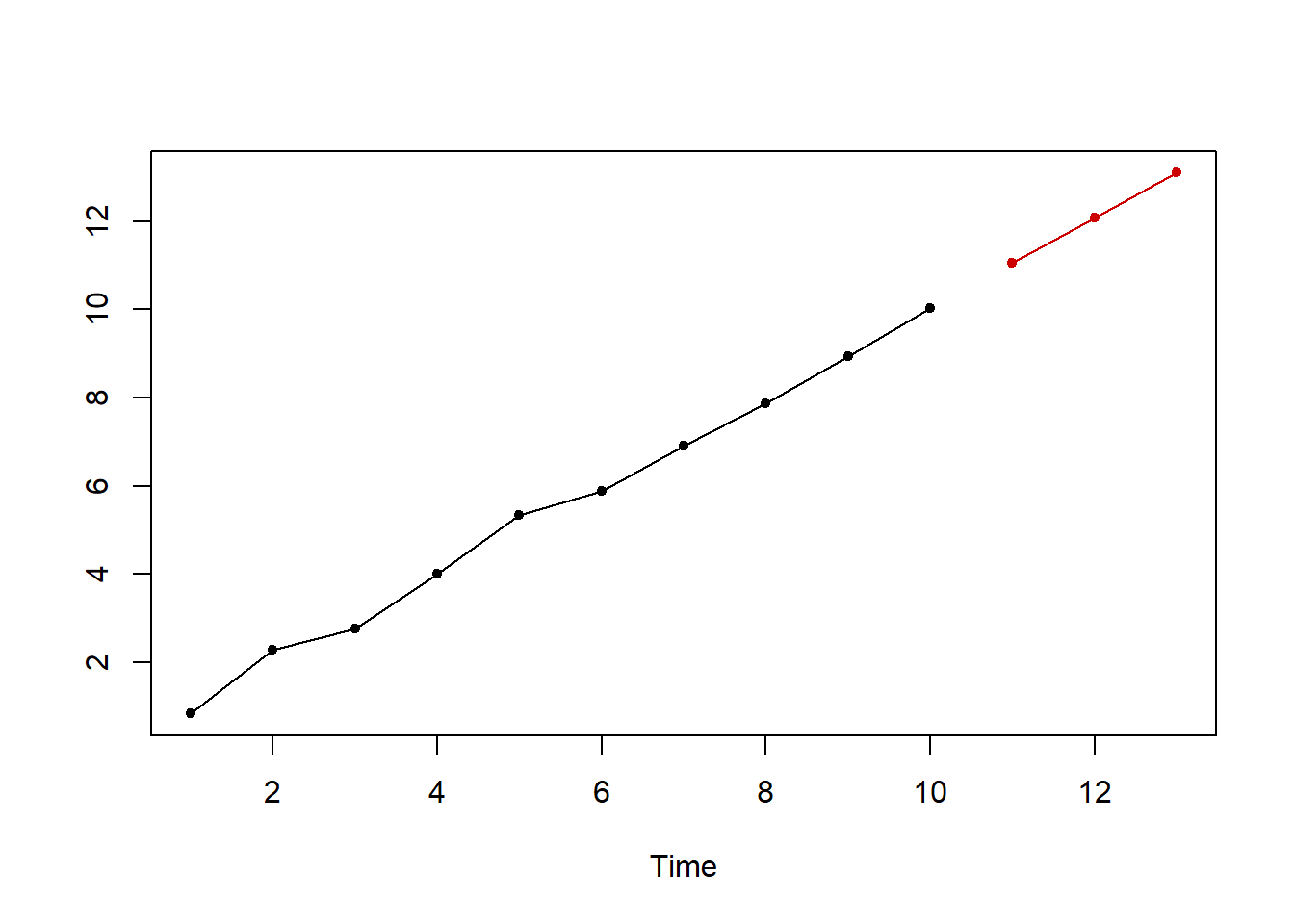## 4.4 Automatic forecasting

Sometimes a great number of time series have to be forecast. In that situation, an automatic way of generating the forecasts is very useful. Our package is able to automatically choose all the KNN parameters. If the user only specifies the time series and the forecasting horizon the KNN parameters are selected as follows:

• As multi-step ahead strategy MIMO is chosen (although the recursive strategy seems to be more effective).
• The combination function used to aggregate the targets is the mean. k* is selected as a combination of three models using 3, 5 and 7 nearest neighbors respectively.
• The lags used as autoregressive features are 1:f, where f is the number of periods of the time series. For example, the lags for quarterly data are 1:4 and for monthly data 1:12. For time series in which the number of periods is one (for example, annual data) the lags with significant autocorrelation in the partial autocorrelation function (PACF) are selected. If no lag has a significant autocorrelation, then lags 1:5 are chosen.
• The additive transformation is applied to the samples, so that a series with a trend can be properly forecasted.

# 5 Evaluating the model

The function rolling_origin uses the rolling origin technique to assess the forecast accuracy of a KNN model. In order to use this function a KNN model has to be built previously. Let us see how rolling_origin works with the following artificial time series:

pred <- knn_forecasting(ts(1:20), h = 4, lags = 1:2, k = 2)
ro <- rolling_origin(pred, h = 4)

The function rolling_origin uses the model generated by a knn_forecasting call to apply rolling origin evaluation. The object returned by rolling_origin contains the results of the evaluation. For example, the test sets can be seen this way:

print(ro$test_sets) ## h=1 h=2 h=3 h=4 ## [1,] 17 18 19 20 ## [2,] 18 19 20 NA ## [3,] 19 20 NA NA ## [4,] 20 NA NA NA Every row of the matrix contains a different test set. The first row is a test set with the last h values of the time series, the second row a test set with the last h - 1 values of the time series and so on. Each test set has an associated training test with all the data in the time series not belonging to the test set. For every training set a KNN model with the parameters associated with the original model is built and the test set is predicted. You can see the predictions as follows: print(ro$predictions)
##      h=1 h=2 h=3 h=4
## [1,]  17  18  19  20
## [2,]  18  19  20  NA
## [3,]  19  20  NA  NA
## [4,]  20  NA  NA  NA

and also the errors in the predictions:

print(ro$errors) ## h=1 h=2 h=3 h=4 ## [1,] 0 0 0 0 ## [2,] 0 0 0 NA ## [3,] 0 0 NA NA ## [4,] 0 NA NA NA Several forecasting accuracy measures applied to all the errors in the different test sets can be consulted: ro$global_accu
## RMSE  MAE MAPE
##    0    0    0

It is also possible to consult the forecasting accuracy measures for every forecasting horizon:

ro$h_accu ## h=1 h=2 h=3 h=4 ## RMSE 0 0 0 0 ## MAE 0 0 0 0 ## MAPE 0 0 0 0 Finally, a plot with the predictions for a given forecast horizon can be generated: plot(ro, h = 4)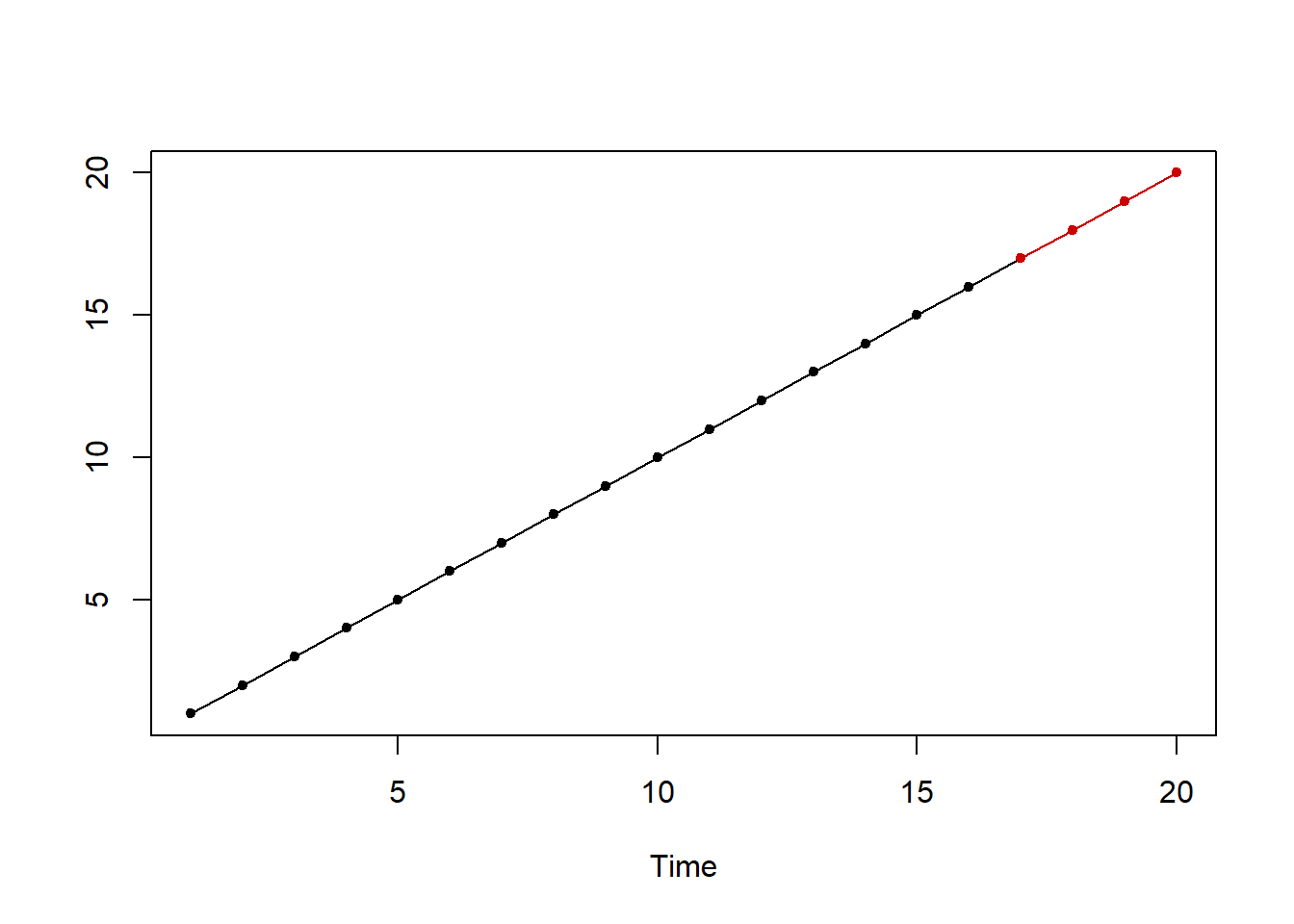The rolling origin technique is very time-consuming, if you want to get a faster assessment of the model you can disable this feature: ro <- rolling_origin(pred, h = 4, rolling = FALSE) print(ro$test_sets)
##      h=1 h=2 h=3 h=4
## [1,]  17  18  19  20
print(ro\$predictions)
##      h=1 h=2 h=3 h=4
## [1,]  17  18  19  20

# 6 Conclusions

In R, just a few packages apply regression methods based on computational intelligence to time series forecasting. In this paper we have presented the tsfknn package that allows to forecast a time series using KNN regression. The interface of the package is quite simple, with only one function the user can specify a KNN model and predict a time series. Furthermore, several graphs can be generated illustrating how the prediction has been computed and the forecasting accuracy of the model can be assessed using hold-out data.